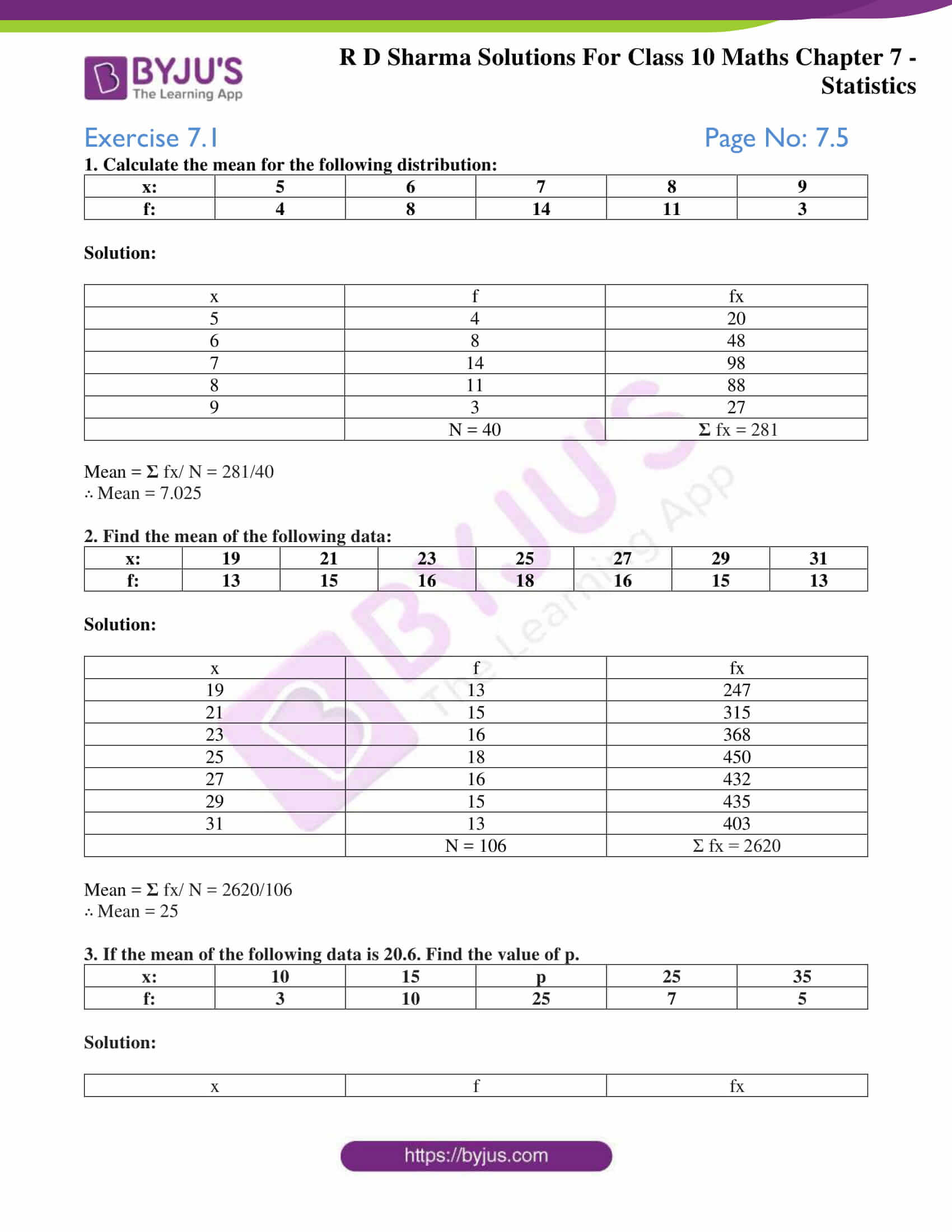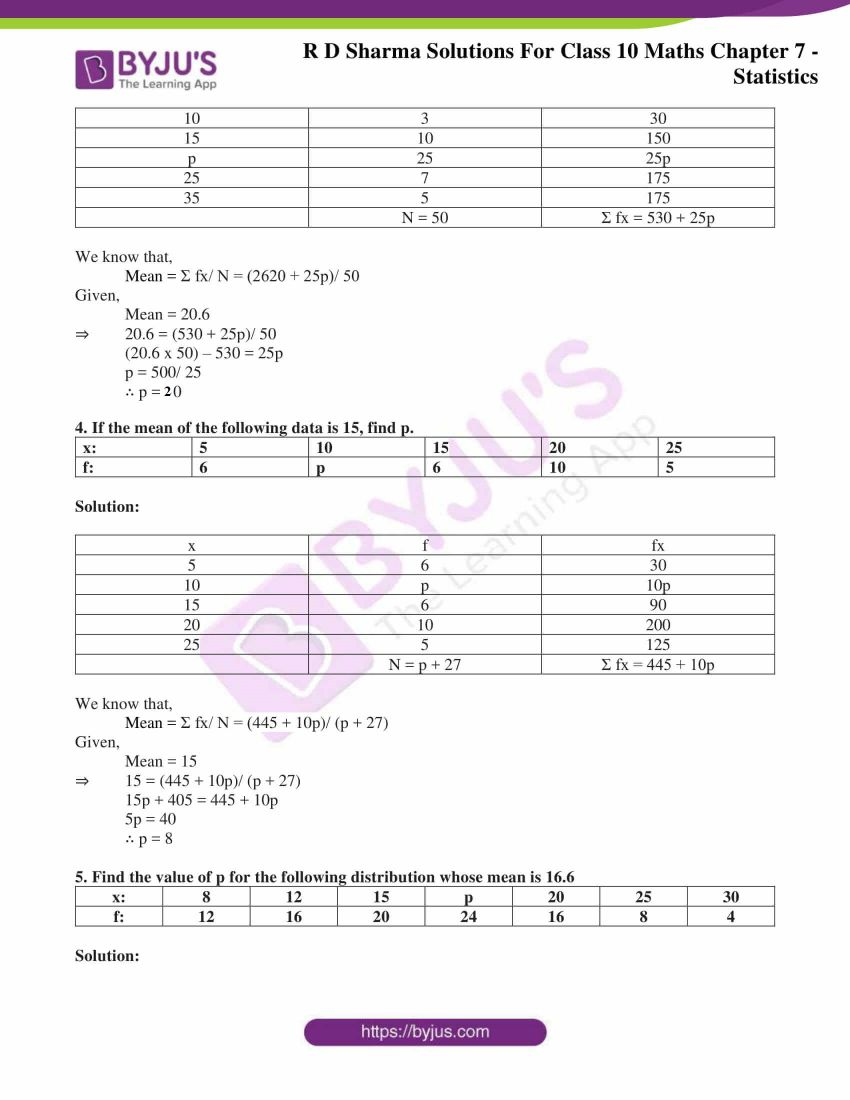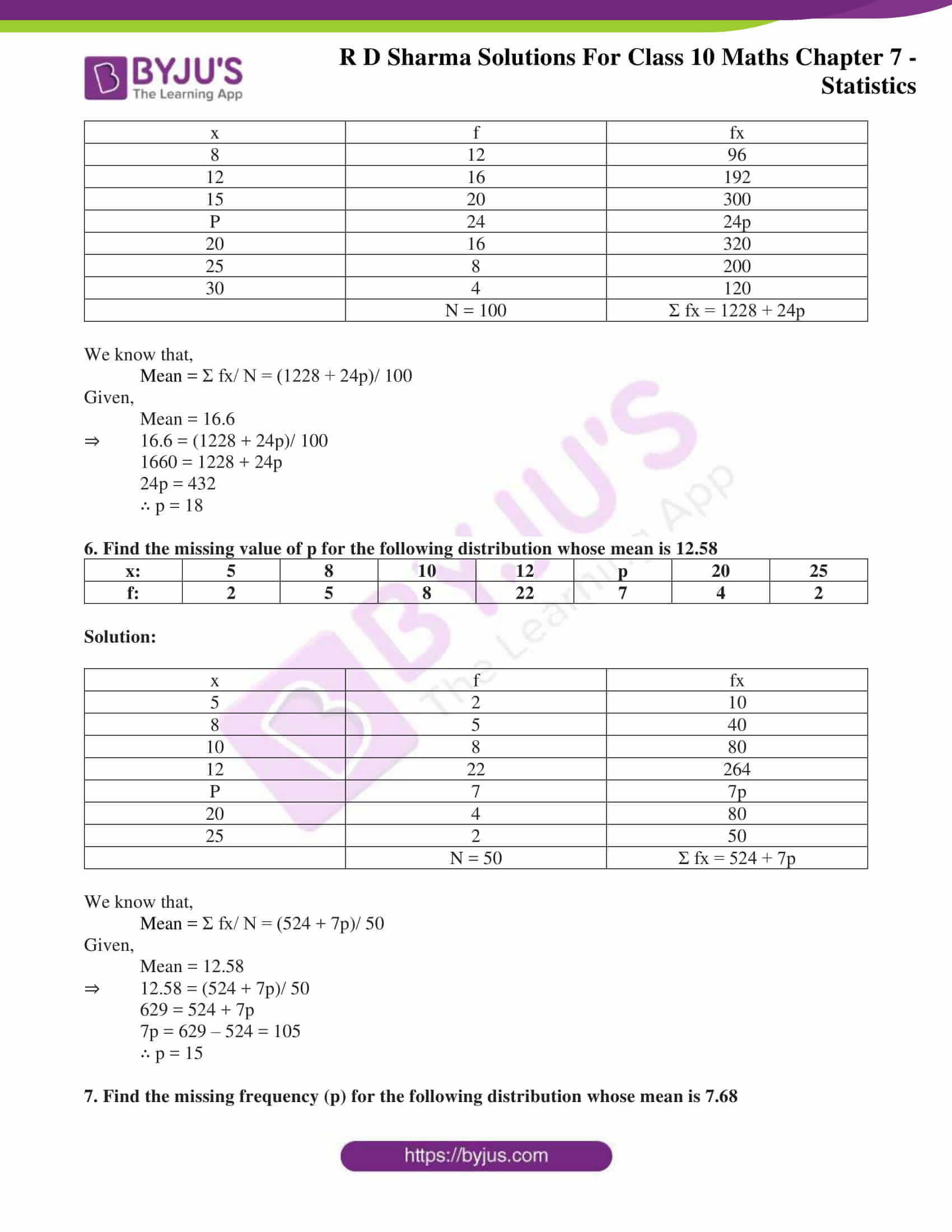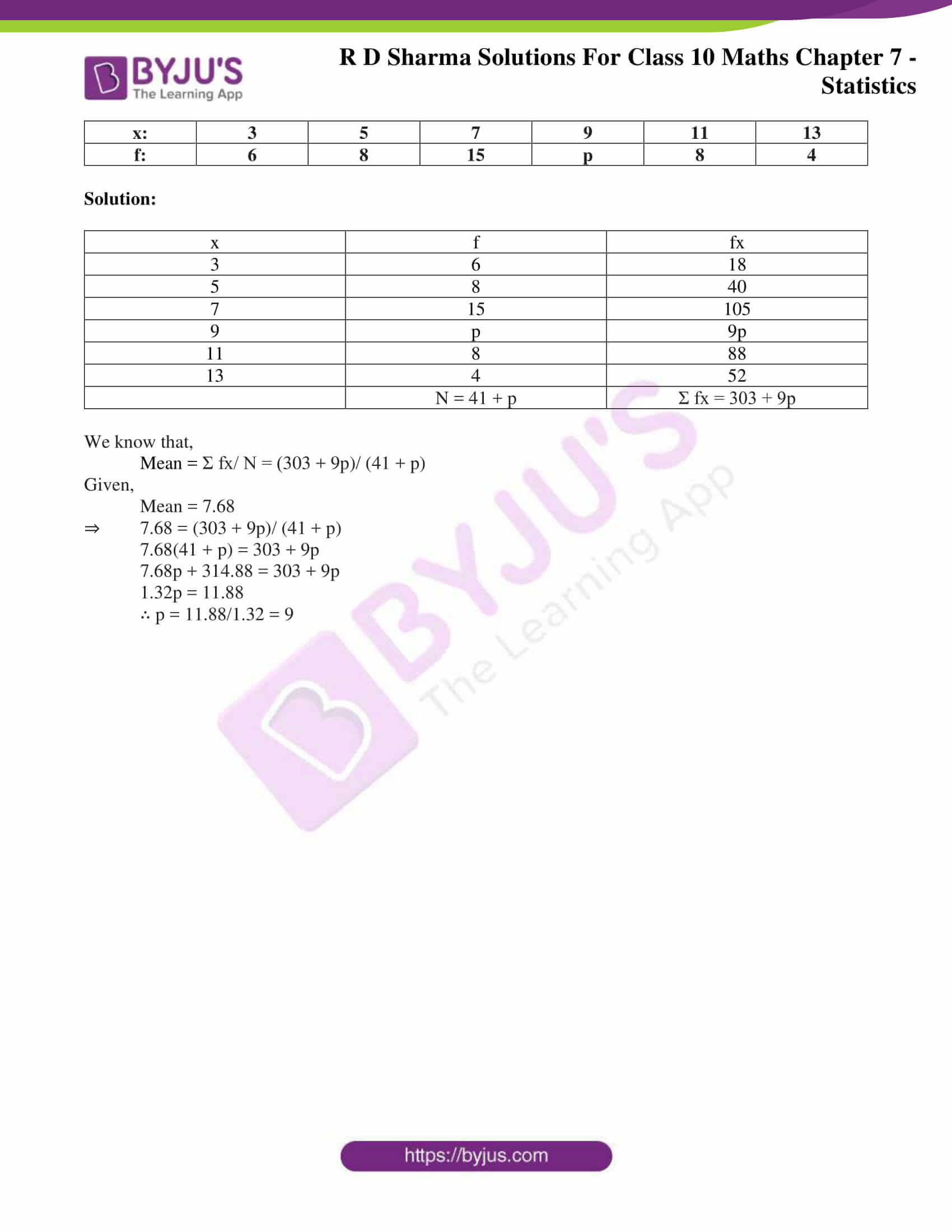# RD Sharma Solutions for Class 10 Maths Chapter 7 Statistics Exercise 7.1

Statistics is purely about data analysis. So, with the given data students will have to find the mean by using different methods. In this exercise, students will have to find the mean of grouped data by using the direct method. The RD Sharma Solutions Class 10 prepared by subject experts at BYJU’S is a very useful resource for students to refer and prepare well for their exams. Download RD Sharma Solutions for Class 10 Maths Chapter 7 Statistics Exercise 7.1, PDF provided below for detailed solutions to problems.

## RD Sharma Solutions for Class 10 Maths Chapter 7 Statistics Exercise 7.1 Download PDF### Access RD Sharma Solutions for Class 10 Maths Chapter 7 Statistics Exercise 7.1

1. Calculate the mean for the following distribution:

 x: 5 6 7 8 9 f: 4 8 14 11 3

Solution:

 x f fx 5 4 20 6 8 48 7 14 98 8 11 88 9 3 27 N = 40 Σ fx = 281

Mean = Σ fx/ N = 281/40

∴ Mean = 7.025

2. Find the mean of the following data:

 x: 19 21 23 25 27 29 31 f: 13 15 16 18 16 15 13

Solution:

 x f fx 19 13 247 21 15 315 23 16 368 25 18 450 27 16 432 29 15 435 31 13 403 N = 106 Σ fx = 2620

Mean = Σ fx/ N = 2620/106

∴ Mean = 25

3. If the mean of the following data is 20.6. Find the value of p.

 x: 10 15 p 25 35 f: 3 10 25 7 5

Solution:

 x f fx 10 3 30 15 10 150 p 25 25p 25 7 175 35 5 175 N = 50 Σ fx = 530 + 25p

We know that,

Mean = Σ fx/ N = (2620 + 25p)/ 50

Given,

Mean = 20.6

⇒ 20.6 = (530 + 25p)/ 50

(20.6 x 50) – 530 = 25p

p = 500/ 25

∴ p = 20

4. If the mean of the following data is 15, find p.

 x: 5 10 15 20 25 f: 6 p 6 10 5

Solution:

 x f fx 5 6 30 10 p 10p 15 6 90 20 10 200 25 5 125 N = p + 27 Σ fx = 445 + 10p

We know that,

Mean = Σ fx/ N = (445 + 10p)/ (p + 27)

Given,

Mean = 15

⇒ 15 = (445 + 10p)/ (p + 27)

15p + 405 = 445 + 10p

5p = 40

∴ p = 8

5. Find the value of p for the following distribution whose mean is 16.6

 x: 8 12 15 p 20 25 30 f: 12 16 20 24 16 8 4

Solution:

 x f fx 8 12 96 12 16 192 15 20 300 P 24 24p 20 16 320 25 8 200 30 4 120 N = 100 Σ fx = 1228 + 24p

We know that,

Mean = Σ fx/ N = (1228 + 24p)/ 100

Given,

Mean = 16.6

⇒ 16.6 = (1228 + 24p)/ 100

1660 = 1228 + 24p

24p = 432

∴ p = 18

6. Find the missing value of p for the following distribution whose mean is 12.58

 x: 5 8 10 12 p 20 25 f: 2 5 8 22 7 4 2

Solution:

 x f fx 5 2 10 8 5 40 10 8 80 12 22 264 P 7 7p 20 4 80 25 2 50 N = 50 Σ fx = 524 + 7p

We know that,

Mean = Σ fx/ N = (524 + 7p)/ 50

Given,

Mean = 12.58

⇒ 12.58 = (524 + 7p)/ 50

629 = 524 + 7p

7p = 629 – 524 = 105

∴ p = 15

7. Find the missing frequency (p) for the following distribution whose mean is 7.68

 x: 3 5 7 9 11 13 f: 6 8 15 p 8 4

Solution:

 x f fx 3 6 18 5 8 40 7 15 105 9 p 9p 11 8 88 13 4 52 N = 41 + p Σ fx = 303 + 9p

We know that,

Mean = Σ fx/ N = (303 + 9p)/ (41 + p)

Given,

Mean = 7.68

⇒ 7.68 = (303 + 9p)/ (41 + p)

7.68(41 + p) = 303 + 9p

7.68p + 314.88 = 303 + 9p

1.32p = 11.88

∴ p = 11.88/1.32 = 9